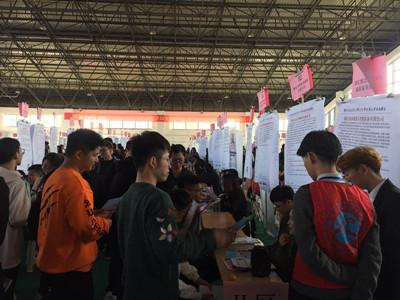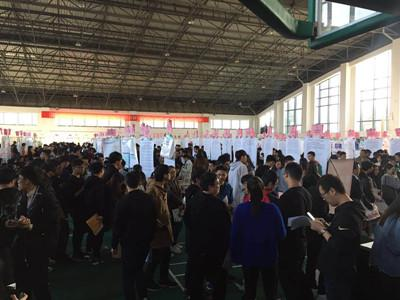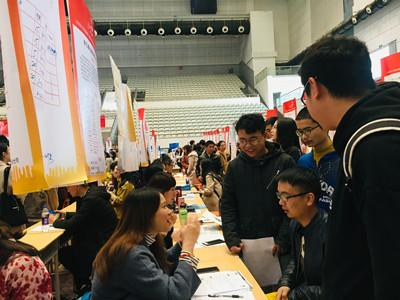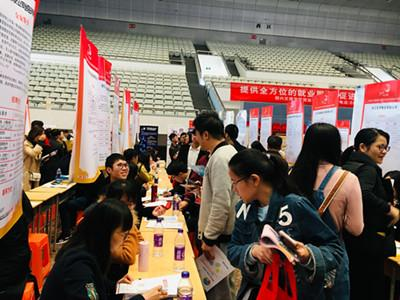#### 请选择工作岗位

• 经营管理类
•     总裁/总经理/CEO
•     技术总监/项目经理/CTO
•     营销总监
•     财务总监/CFO
•     人力资源总监
•     行政总监
•     经理助理
•     企管部经理/管理顾问
•     企业管理人员
•     信息主管CIO
•     投资管理
•     厂长/副厂长
• 计算机/IT业人员
•     系统分析员
•     软件（测试）工程师
•     硬件（测试）工程师
•     网络工程
•     网站信息编辑
•     网站策划
•     网页设计/电脑美工
•     多媒体设计与开发
•     计算机辅助设计与绘图
•     数据库开发与管理
•     系统集成/支持
•     系统安全管理
•     Internet/WEB/电子商务
• 电子/通讯类专业人员
•     电子工程师
•     电子元器件工程师
•     电子/电器维修
•     无线电技术
•     电路设计/电子测试/半导体技术
•     通讯工程师
•     自动控制
•     单片机/DLC/DSP/底层软件开发
•     仪器/仪表/计量
•     智能大厦/综合布线/弱电
•     电气
•     电力
• 销售/业务类
•     销售经理/主任
•     销售主管/代表
•     销售助理
•     商务经理/主管
•     商务代表
•     涉外业务
•     促销员
• 市场营销/公关
•     市场/营销经理
•     市场助理/专员
•     产品/品牌企划
•     市场通路企划
•     价格企划
•     广告企划
•     新闻媒介策划
•     市场推广/拓展/合作
•     市场分析/调研
•     公关经理/主管
•     公关专员
•     广告创意/设计/策划
• 客户服务类
•     客户服务经理
•     客户培训
•     客户关系管理
•     售前/售后支持
•     客户数据库维护
•     客户分析
•     客户渠道
•     投诉处理
•     投诉监控
•     热线人员
• 行政/人力资源管理类
•     行政经理
•     行政主管/助理
•     办公室主任
•     行政秘书/行政专员
•     人力资源经理
•     招聘经理/主管/专员
•     培训经理/主管/专员
•     薪酬福利经理/主管/专员
•     绩效考核经理/主管/专员
•     人事主管/助理/专员
• 文职类
•     图书情报/资料/文档管理
•     资料/文档的撰写/编辑
•     文秘/高级文员
•     文员/电脑录入/校对
•     前台/接待/礼仪
•     热线咨询
• 翻译类
•     英语
•     日语
•     法语
•     德语
•     朝鲜语
•     西班牙语
•     俄语
• 财务/审计/统计
•     财务经理/副经理/主任
•     会计师
•     注册会计师
•     会计主管
•     会计
•     财务会计
•     管理会计
•     出纳
•     审计经理/主管/专员
•     统计
• 设计类
•     美术/图形设计
•     工业/产品设计
•     工艺品设计
•     室内外装修/装潢设计
•     纺织服装设计
•     家具/珠宝设计
•     形象设计
•     电脑绘图
• 工业/工厂类
•     生产管理
•     工程管理
•     品质管理
•     物料管理
•     物流管理
•     设备管理
•     采购管理
•     仓库管理
•     计划/调度员
•     化验/检验员
•     跟单/单证员
•     PMC/SMT技术员
• 金融/经济专业人员
•     金融/投资
•     信贷/证券
•     期货经济
•     外汇
•     资产评估
•     税务经理/主管/专员
•     保险业务/经纪人
•     预结算人员
•     稽核员
•     报关员
•     船务
• 法律专业人员
•     律师
•     法律顾问
•     法务助理
• 机械专业人员
•     机械工程师
•     模具工程师
•     机电工程师
•     机械设计
•     机械制图
• 房地产/土建类专业人员
•     房地产开发/策划
•     房地产评估/交易
•     建筑/结构工程师
•     工程监理师
•     工程预决算
•     给排水/水电工程师
•     水电管理
•     建筑施工管理
•     制冷暖通
•     物业管理
•     工民建
•     路桥工程
•     管道
•     绘图/建筑制图
•     基础地下工程/岩土工程
•     港口与航道工程
• 咨询/顾问
•     咨询经理
•     咨询员
•     专业顾问
•     信息中介
•     培训师
• 医疗/护理/保健类
•     中/西医师
•     医药技术员
•     护士/护理人员
•     医/药学检验员
•     兽医/宠物医生
•     心理医生
•     药剂师
•     麻醉师
•     卫生防疫
•     妇幼保健
•     针灸推拿
•     化妆/美容师
•     化工制药
• 技工类
•     钳工/机修工/钣金工
•     电焊工/铆焊工
•     车工/磨工/铣工/冲床/锣床/车床
•     模具工
•     水工/木工/油漆工
•     电工
•     叉车工
•     空调工/电梯工/锅炉工
•     裁剪车缝熨烫
•     普工
• 文教体
•     新闻采编/记者/摄影
•     编辑
•     出版
•     高等教育
•     中等教育
•     小学/幼儿教育
•     职业教育/家教
•     体育类
•     影视策划/制作
•     表演人员
•     节目主持/DJ/播音
•     音效师
• 服务业专业人员
•     旅游/导游
•     宾馆酒店
•     娱乐餐饮
•     寻呼/声讯
•     收银员/营业员/服务员
•     理货员
•     司机
•     保安
• 纺织印染
•     家用纺织品
•     面料
•     纺织机械及器材
•     纤维原料
•     染料助剂
•     纱线
•     服装服饰
•     皮革及皮毛
•     无纺布
•     辅料
•     印染及后整理服务
•     其他
•     设计师
•     设计总监
•     产品总监
•     跟单员
•     制版师
•     样板师
•     工艺师
•     市场总监
•     销售主管
•     销售助理
•     生产厂长
•     车间主任
•     生产管理
•     采购主管
•     高级技师
•     外贸人员
•     印染工程师
•     行政人员
•     人力资源
•     财务/统计
•     翻译
•     单证员
•     技工
• 实习生

• 浙江
• 浙江/绍兴市
• 浙江/杭州市
• 浙江/宁波市
• 浙江/金华市
• 浙江/台州市
• 浙江/衢州市
• 浙江/温州市
• 浙江/嘉兴市
• 浙江/湖州市
• 浙江/舟山市
• 浙江/丽水市
• 北京
• 天津
• 河北
• 山西
• 内蒙古
• 辽宁
• 吉林
• 黑龙江
• 上海
• 江苏
• 安徽
• 福建
• 江西
• 山东
• 河南
• 湖北
• 湖南
• 广东
• 广西
• 海南
• 四川
• 贵州
• 云南
• 西藏
• 陕西
• 甘肃
• 青海
• 宁夏
• 新疆
• 其他
• 重庆

#### 请选择发布日期

• 3天内
• 半月内
• 一个月内
• 一周内
• 三个月内
• 半年内

 ZJ1078026 硕士 建筑与土木工程 ZJ1077991 大专 纺织装饰艺术设计 ZJ1077976 本科 电子信息工程 ZJ1077974 本科 酒店管理 ZJ1077971 本科 电子信息科学与技术 ZJ1077917 本科 信息安全 ZJ1077906 本科 环境设计 ZJ1077902 本科 会计学 ZJ1077901 本科 戏剧影视文学 ZJ1077887 本科 会计学 ZJ1077881 本科 会计学 ZJ1077867 本科 会计 ZJ1077857 本科 会计（专）人力资源 ZJ1077847 本科 会计学 ZJ1077840 本科 市场营销

2018年柯桥区“冬季招才校园行”火热进行